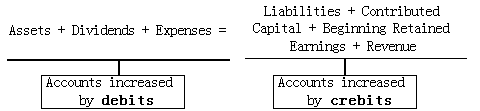### Why should I choose AnalystNotes?

Simply put: AnalystNotes offers the best value and the best product available to help you pass your exams.

### Subject 3. Accounting Equations

Assets reported on the balance sheet are either purchased by the company or generated through operations; they are financed, directly or indirectly, by the creditors and stockholders of the company. This fundamental accounting relationship provides the basis for recording all transactions in financial reporting and is expressed as the balance sheet equation:

Assets (A) = Liabilities (L) + Owners' equity (E)

This equation is the foundation for the double-entry bookkeeping system because there are two or more accounts affected by every transaction.

If the equation is rearranged:

Assets - Liabilities = Owners' equity

The above equation shows that the owners' equity is the residual claim of the owners. It is the amount left over after liabilities are deducted from assets.

Owners' equity at a given date can be further classified by its origin: capital provided by owners, and earnings retained in the business up to that date.

Owners' equity = Contributed capital + Retained earnings

Net income is equal to the income that a company has after subtracting costs and expenses from total revenue.

Revenue - Expenses = Net income (loss)

Net income is informally called the "bottom line" because it is typically found on the last line of a company's income statement.

Balance sheets and income statements are interrelated through the retained earnings component of owners' equity.

Ending retained earnings = Beginning retained earnings + Net income - Dividends

The following expanded accounting equation, which is derived from the above equations, provides a combined representation of the balance sheet and income statement:

Assets = Liabilities + Contributed capital + Beginning retained earnings + Revenue - Expenses - Dividends

• Dividends and expenses decrease owners' equity.
• Revenues increase owners' equity.

Because dividends and expenses are deductions from owners' equity, move them to the left side of the equation:#### Practice Question 1

At the beginning of the year, Jeeze Corporation had assets of \$85,000 and stockholders' equity of \$40,000. If assets increased by \$30,000 and liabilities increased by \$35,000, what was the stockholders' equity at the end of the year?

A. \$5,000
B. \$35,000
C. \$45,000

Using the accounting equation: Assets = Liabilities + Stockholders' Equity, liabilities were \$45,000 at the beginning of the year (\$85,000 = \$45,000 + \$40,000). During the year, assets increased by \$30,000 to \$115,000 and liabilities increased by \$35,000 to \$80,000. Using the accounting equation again, stockholders' equity is determined to be \$35,000 at year-end (\$115,000 = \$80,000 + \$35,000).

#### Practice Question 2

True or False? One way of stating the accounting equation is Assets + Liabilities = Stockholders' Equity.

The accounting equation is Assets = Liabilities + Stockholders' Equity. It states that the assets held by a business are equal to the claims against those assets. The claims against the assets are the owners' claims (stockholders' equity) and claims of creditors for outstanding loans to the business (liabilities). The equation may be restated as Assets - Liabilities = Stockholders' Equity.

#### Practice Question 3

Which of the following statements is true?

I. Assets = Liabilities + Common Stock + Retained Earnings - Dividends - Revenues + Expenses
II. Assets + Dividends + Revenues = Liabilities + Common Stock + Retained Earnings + Expenses

Assets = Liabilities + Common Stock + Beginning Retained Earnings - Dividends + Revenues - Expenses

Also, Assets + Dividends + Expenses = Liabilities + Common Stock + Beginning Retained Earnings + Revenues

#### Practice Question 4

An analyst gathers the following information from a company's accounting records (all figures in thousands):

Assets, 31 December 2013: \$5,250
Liabilities, 31 December 2013: 2,200
Contributed capital, 31 December 2013: 1,400
Retained earnings, 1 January 2013: 800
Dividends declared during 2013: 200

The analyst's estimate of net income (\$ thousands) for 2013 is closest to:

A. 850
B. 950
C. 1,050

Total assets = liabilities + owner's equity
Owner's equity = \$5,250 - 2,200= 3,050
Owners equity = contributed capital + ending retained earnings
Ending retained earnings = 3,050 1,400= 1,650
Ending retained earnings = beginning retained earnings + net income - dividends
1,650= 800 + net income - 200
Net income = \$1,050

#### Practice Question 5

The following information is available for a company:

December 31, 2013:
Total Assets: \$100,000
Net income for the year: \$4,000
Dividends paid: \$0
Assets are equally financed with debt and equity
50% of the equity comes from contributed capital

December 31, 2014:
Total Assets: \$92,000
Net loss for the year: \$3,000
No new debt or equity issued or repurchased

In 2014, the company most likely ______

A. paid a dividend of \$1,000.
B. paid a dividend of \$5,000.
C. did not pay a dividend because they incurred a loss.